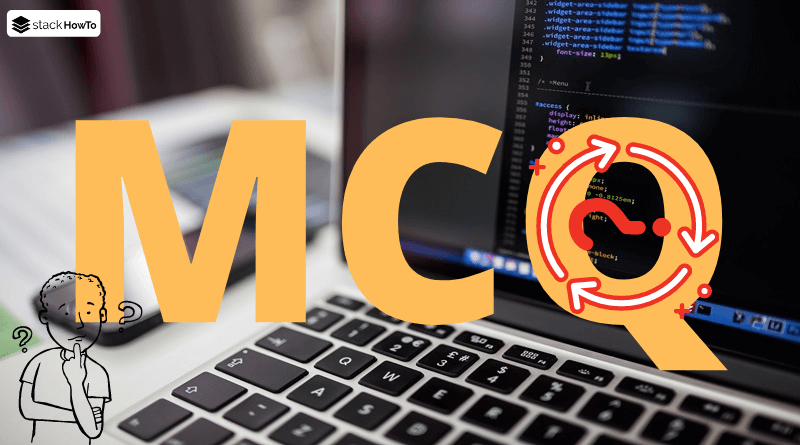MCQ

# Java MCQ – Multiple Choice Questions and Answers – Strings – Part 1

This collection of Java Multiple Choice Questions and Answers (MCQs): Quizzes & Practice Tests with Answer focuses on “Java Strings”.

###### 1. Which of the following class is the superclass of String and StringBuffer class?

A ArrayList

B java.util

C java.lang

D java.string

C
String and StringBuffer classes belong to java.lang package.

###### 2. Which of the following operators, we can use to concatenate two String?

A ||

B &

C +=

D +

D
We can use the + operator to concatenate two String. Example:

```String s = "Welcome" + " To" + "StackHowTo!";
System.out.println(s); //Welcome To StackHowTo!```

###### 3. String in Java is a _________?

A object

B class

C reference

D array of character

B
String in Java is a class.

###### 4. What is the output of the following code?
```public class Q4
{
public static void main(String []args)
{
String s1 = new String("Ali");
String s2 = new String("Alis");
System.out.println(s1 = s2);
}
}```

A true

B false

C Ali

D Alis

D
String s2 is assigned to s1. So now s2 (Alis) is now present in s1 and prints “Alis”

###### 5. Which of the following method is used to get the length of a String object?

A getSize()

B Sizeof()

C len()

D length()

D
length() is used to get the length of a String object. Example:

```String str = new String("Ali");
System.out.println(str.length()); //3```

###### 6. Which of the following method is used to get the character at specified index?

A getChar()

B Charat()

C charat()

D charAt()

D
charAt() is used to get the character at specified index. Example:

```String str = new String("Ali");
char c = str.charAt(0);
System.out.println(c); //A```

###### 7. What is the output of the following code?
```public class Q7
{
public static void main(String []args)
{
String s1 = new String("Hello");
String s2 = new String(s1);
System.out.println(s1 == s2);
}
}```

A Hello

B true

C false

D Error

C
When we use the “new” keyword new string will be created in heap area. if we compare s1 and s2 using == operator it will compare references, here references is not identical so it will return false.

###### 8. Which of the following method is used to test tow strings for equality?

A checkEqual()

B eq()

C equals()

D equal()

C
equals() method is used to test tow strings for equality.

###### 9. What is the output of the following code?
```public class Q8
{
public static void main(String []args)
{
String s1 = "Hello";
String s2 = "Hello";
System.out.println(s1.equals(s2));
System.out.println(s1 == s2);
}
}```

A false true

B true false

C false false

D true true

D
• s1.equals(s2) checks for values so s1 and s2 are identical, so it’s true.
• s1 == s2 checks for references here we haven’t used “new” keyword so references of s1 and s2 are identical, so it’s true.

###### 10. Which of the following affirmations are incorrect?

A Each string is an object of class String

B Strings in java are changeable

C String is a class

D Java defines a fellow class of String, called StringBuffer, which enables string to be modified

B
Strings in Java are not changeable, so they can not be modified. Example:

```String str = new String("ABC");
str.concat("DEF");
System.out.println(str);```

Output:

`ABC`

The value of string str is not updated due to strings are immutable.MCQPractice competitive and technical Multiple Choice Questions and Answers (MCQs) with simple and logical explanations to prepare for tests and interviews.Read More